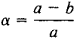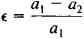# Oblateness of the Earth

The following article is from The Great Soviet Encyclopedia (1979). It might be outdated or ideologically biased.

## Oblateness of the Earth

a quantity that characterizes the degree to which the earth is flattened in the direction of its axis of rotation, that is, the deviation of the earth’s shape from that of a sphere. The polar oblateness of the earth α is expressed by the equationwhere a is the equatorial radius of the earth and b is the polar radius. According to modern findings, α = 1:298.3. With the discovery that the earth is also flattened at the equator, the concept of the equatorial oblateness of the earth was introduced:where a1 and a2 are the maximum radii of the earth’s equator. According to available data, ∊ = 1:30,000, and the difference between a1 and a2 is approximately 210m.